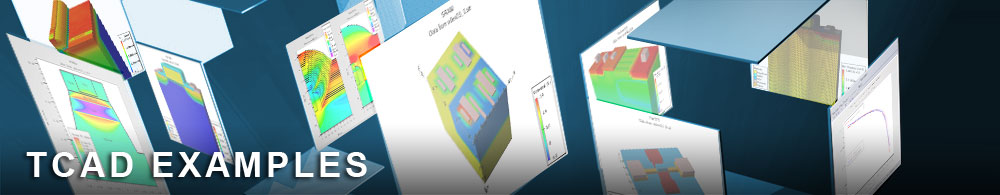CV Analysis Of Thin Gate Oxide PMOS Capacitor

## quantumex01.in : CV Analysis Of Thin Gate Oxide PMOS Capacitor

Requires: S-Pisces/Quantum
Minimum Versions: Atlas 5.28.1.R

This example demonstrates the CV characteristics of a N-type substrate MOS capacitor with a nominal 30A gate oxide. The example shows:

• Calculation of CV curves using AC Analysis
• Use of the Quantum moments quantum model
• Difference between classical and quantum gate thickness
• Classical and Quantized electron density

A simple 1D MOS capacitor is created in this example with a 30A gate oxide thickness. An AC analysis is performed on the device firstly with the classical simulation and the with the quantum model swtiched on.

The quantum model is activated by using the quantum switch in the models statement for electrons. Note that this activates a quantum moments model which uses a quantum temperature calculation. This quantum temperature can be viewed in the structure files. Also, the cutline created in TonyPlot can be used to examine the classical and quantum electron concentration differences in the structure.

In accumulation, the channel carrier concentration is changed by the addition of quantum mechanics. The peak is not so high and the electrons spread more deeply into the substrate. The difference in gate capacitance is noticable on the CV plot. Since gate oxide thickness is often measured using CV techniques, this leads to erroneous results if QM effects are not accounted for. extract statements are used to show the calculated Tox from the classical and QM CV curves.

To load and run this example, select the Load button in DeckBuild > Examples. This will copy the input file and any support files to your current working directory. Select the Run button in DeckBuild to execute the example.